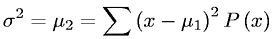Equations > Statistics > Moments > Variance or second moment about the Mean

### Variance or second moment about the MeanLatex Code:

MathML Code:

 ${\sigma }^{2}={\mu }_{2}=\sum {\left(x-{\mu }_{1}\right)}^{2}P\left(x\right)$

MathType 5.0: